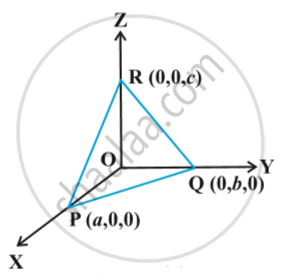# Plane - Intercept Form of the Equation of a Plane

## Notes

the equation of a plane in terms of the intercepts made by the plane on the coordinate axes.  Let the equation of the plane be
Ax + By +Cz +D = 0      (D ≠ 0)                      ... (1)
Let the plane make intercepts a, b, c on x, y and z axes, respectively in following fig.Hence, the plane meets x, y and z-axes at (a, 0, 0), (0, b, 0), (0, 0, c), respectively.
Therefore  Aa + D = 0  or A = (-D)/a
Bb + D = 0   or B = (-D)/b
Cc +D = 0  or C = (-D)/c
Substituting these values in the equation (1) of the plane and simplifying, we get
x/a + y/b +z/ c = 1                    ...(1)

If you would like to contribute notes or other learning material, please submit them using the button below.

### Shaalaa.com

3 Dimensional Geometry part 21 (Plane in intercept form) [00:07:12]
S
0%